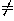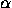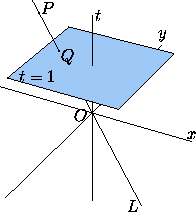# 1.4 Homogeneous Coordinates in the Plane

A triple of real numbers (x:y:t), with t0, is a set of homogeneous coordinates for the point P with cartesian coordinates (x/t, y/t). Thus the same point has many sets of homogeneous coordinates: (x:y:t) and (x':y':t') represent the same point if and only if there is some real numbersuch that x'=x, y'=y, t'=t. If P has cartesian coordinates (x,y), one set of homogeneous coordinates for P is (x,y,1).

When we think of the same triple of numbers as the cartesian coordinates of a point in three-dimensional space (Section 9.1), we write it (x,y,t) instead of (x:y:t). The connection between the point in space with cartesian coordinates (x,y,t) and the point in the plane with homogeneous coordinates (x:y:t) becomes apparent when we consider the plane t=1 in space, with cartesian coordinates given by the first two coordinates x, y of space (Figure 1). The point (x,y,t) in space can be connected to the origin by a line L that intersects the plane t=1 in the point with cartesian coordinates (x/t, y/t), or homogeneous coordinates (x:y:t).Figure 1: The point P with spatial coordinates (x,y,t) projects to the point Q with spatial coordinates (x/t, y/t, 1). The plane cartesian coordinates of Q are (x/t, y/t), and (x:y:t) is one set of homogeneous coordinates for Q. Any point on the line L (except for the origin O) would also project to P'.

Projective coordinates are useful for several reasons, one the most important being that they allow one to unify all symmetries of the plane (as well as other transformations) under a single umbrella. All these transformations can be regarded as linear maps in the space of triples (x:y:t), and so can be expressed in terms of matrix multiplications. (See Section 2.2).

If we consider triples (x:y:t) such that at least one of x, y, t is nonzero, we can name not only the points in the plane but also points ``at infinity''. Thus (x:y:t) represents the point at infinity in the direction of the line with slope y/x.

Next: 1.5 Oblique Coordinates in the Plane
Up: 1 Coordinate Systems in the Plane
Previous: 1.3 Polar Coordinates in the PlaneThe Geometry Center Home Page

Silvio Levy
Wed Oct 4 16:41:25 PDT 1995

This document is excerpted from the 30th Edition of the CRC Standard Mathematical Tables and Formulas (CRC Press). Unauthorized duplication is forbidden.